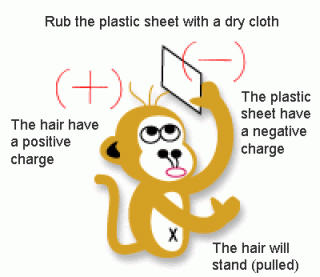## Wednesday, May 25, 2011

### Electrostatic1. Underline the correct answer below to describe what electrostatics is.
(Electrostatic / Electricity) is the study of static electrical charges.
2. Underline the correct answer below to state the types of static electrical charges
a. Objects with the same type of charges (repel / attract) each other.
b. Objects with different types of charges (attract / repel) each other.
3. Underline the correct answer below to state the properties of static electrical charges.
a. A positively-charged object has more (protons / electrons) than (protons / electrons).
b. A negatively-charged object has more (protons / electrons) than (protons / electrons).
c. A (positively charge / neutral) object has the same number of protons and electrons.

## Sunday, May 22, 2011

### CHAPTER 7: ELECTRICITY7.1 Electrostatics

1. Electrostatics is the study of static electrical charges.

2. There are two types of electric charge: positive charge and negative charge.

3. Positive charges consist of protons and negative charges consist of electrons.

4. A neutral object has the same number of positively and negatively charged
particles.

5. An object that loses electrons becomes positively charged because there are
more protons than electrons.

6. The transfer of charged particles happens when two different substances are
rubbed. The charge formed by friction is known as electrostatic charge.

7. The Detection of Electrostatic Charge

Electrostatic charge can be detected by using an electroscope.

7.2 Electricity
1. Some sources of electrical energy are the solar cell, dry cell, wet cell, power generator, lithium ion battery and cadmium battery.
2. An electric current (I), is defined as the rate of flow of electrons.

3. The electrical force needed to move electrons between two points is called the
voltage (V) or potential difference between the two points.

4. The property of a materials that prevents or resists the flow of electrons
through the material is called the resistance (R).

7.3 Electric Current, Voltage and Resistance

 Electrical Current Voltage Resistance Definition Quantity of electric charge that flows through a conductor in The potential difference between two points in an electric circuit The opposition to the flow of electric charge Measuring instrument Ammeter Voltmeter Using Ohm's Law R = V I Symbol I V R Method of connection Series Parallel Resistance of wire depends on: • length • diameter • type of metal

7.4 Relationship between current, voltage and resistance

1. Ohms Laws states that the current flowing through a conductor is directly
proportional to its voltage.

2. Ohm’s law is given by the following formula,

7.5 Parallel and Series Circuits
 Series circuit Difference in term Parallel circuit The electrical components are connected end to end Method of connection The electrical components are connected side by side The current flows in one channel only Number of channels The current flows in more than one channel The other bulbs will not light up If one bulb burns out The other bulbs will still light up Same in every resistance I = I1 = I2 = I3 Amount of current Same as the total amount of current in every channel I=I1 + I2 + I3 Same as the amount of voltage through every bulb V = VI + V2 + V3 Amount of voltage Voltage is the same as the voltage supply V1 = V2 = V3 = V Total resistance (R) is the same as total of all resistances R=RI+R2+R3 Amount of resistance Total resistance (R) is calculated as follows: 1 = 1 + 1 + 1 R RI R2 R3 Does not last long Lifespan Lasts long Increases if the number of cells increases Current Same even though the number of cells increases

7.6 Magnetism

1. Magnetism is a group of phenomena associated with magnetic fields.

2. The characteristics of a magnet are:

(a) A magnet has two poles the north and the south poles.
(b) The same poles repel, different poles attract each other.

(c) A free hanging magnet always points in the north-south direction.
(d) A magnet can attract iron, steel, cobalt and nickel.

3. A magnetic field is a field of force surrounding a magnetic body.

7.7 Electromagnetism

1 An electromagnet is a temporary magnet formed when an electric current is passed through a coil of conductor wire.

2 The current that flows through the conductor produces a magnet field around it

3 The direction of the magnetic field of a straight wire can be determined by using the

RIGHT HAND GRIP RULE.

a. The thumb represents the direction of the current flow

b. The curved fingers represent the direction of the magnetic field

4. The strength of a solenoid magnetic field can be increased by

a. increasing the number of turns in the coiled wire.
b. Inserting a laminated iron bar into the solenoid.

c. Increasing the current flow.

5. Electromagnets are used in loud speakers, electric bells, telephone receivers and telegraph machines.

6. The strength of a solenoid magnetic field can be increased by

d. increasing the number of turns in the coiled wire.
e. Inserting a laminated iron bar into the solenoid.

f. Increasing the current flow.